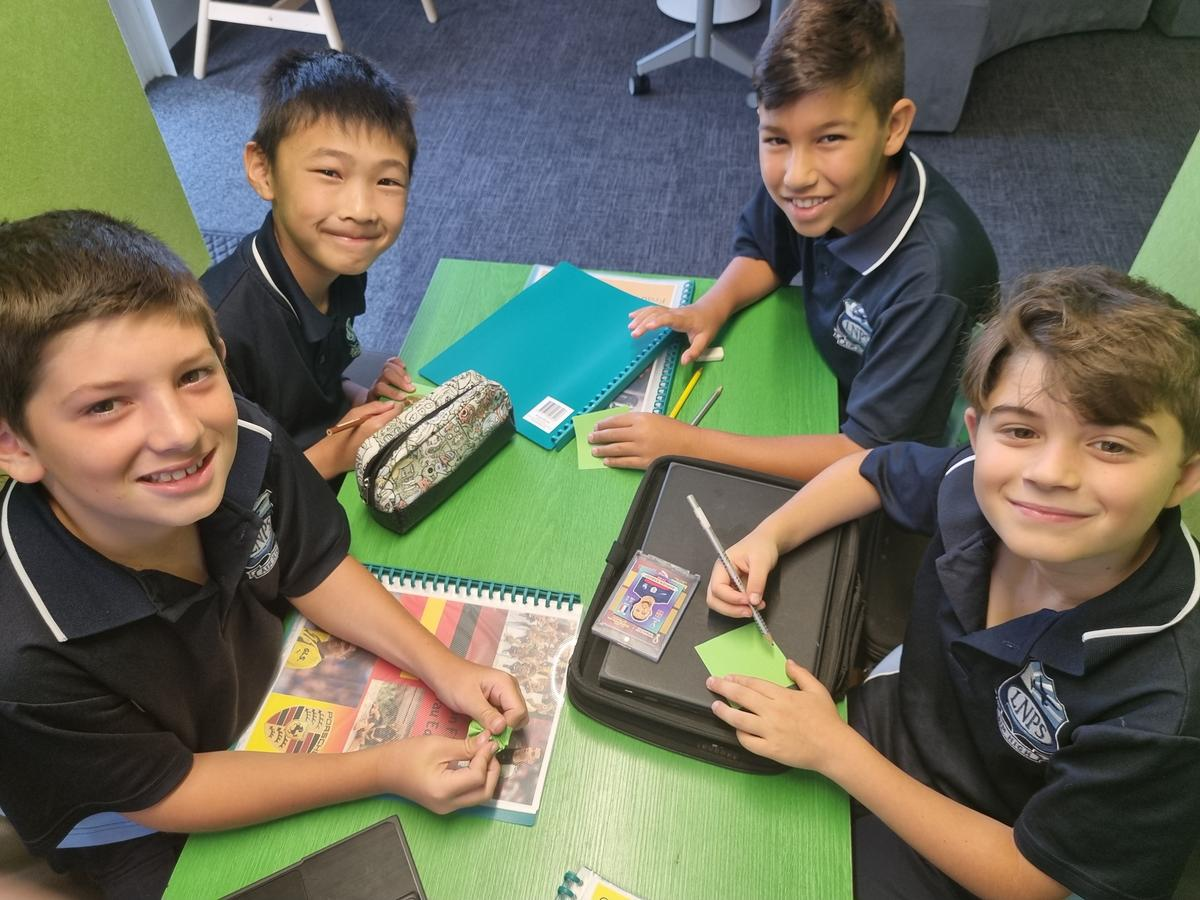# MATHS

## Number and Money

Learning intentions

Students will:

• understand that the groupings of hundreds, tens, ones, tenths and so on can be taken apart in different but equivalent ways
• know that the place value system extends infinitely in 2 directions
• recognise, represent, and order numbers to at least tens of thousands
• partition, rearrange and regroup numbers to at least tens of thousands
• understand that decimals are a way of recording fractions within the base-ten system
• make connections between fractions and decimals
• solve problems involving the calculation of money to the nearest 5c.

## Number: Multiplication, division and odd and even numbers

Learning intentions

Students will:

• identify that an even number is divisible by 2 and will use the properties of odd and even numbers with all 4 operations
• know that number sequences and their patterns can be extended indefinitely by investigating number sequences and their patterns
• understand that multiplication involves counting groups of equal sizes and determining how many there are in total or, using a representative set as unit in a
• multiplicative comparison
• recall multiplication facts up to 10 × 10 and use these facts to recall related division facts
• understand that division is the inverse of multiplication and that multiplication involves combining equal sets and division involves sharing into equal sets
• represent division as sharing into equal sets, they will recognise the ÷ symbol
• choose efficient mental and written strategies to solve multiplication and division problems with no remainder.

## Properties of prime, composite, square and triangular numbers, negative and positive integers, computational strategies

Learning intentions

Students will:

• recall number properties. They will identify the properties of prime numbers. They will investigate composite numbers and find factor pairs. They will understand that rows and the columns in an array represent two factors of a given number.
• represent factors by making arrays. They will use critical and creative thinking by applying properties of operations to generate equivalent expressions.
• explore the properties of prime and composite numbers. They will investigate whether a number is prime or composite by finding factors. They will connect the common multiples of a number.
• decompose numbers into prime factors. They will understand that composite numbers are built up from prime factors. They will model how to record index notation. They will use critical and creative thinking to communicate solutions effectively.
• identify, describe and record properties of square numbers. They will apply index notation to square numbers to model efficient expressions. They will use critical and creative thinking to partition square numbers and produce equivalent expressions.
• identify, describe and record properties of triangular numbers
• identify everyday situations that use positive and negative integers. They will plot positive and negative integers on a number line. Students will locate and represent negative and positive numbers on a number line and solve everyday problems involving integers.

## Patterns and Algebra

Learning intentions

Students will:

• explore the concept of patterns involving regularity and explain how this regularity changes depending on the pattern type.
• identify missing values from number patterns including integers, fractions, and decimals.
• explain the additive and multiplicative rules to an arithmetic or geometric pattern.
• create arithmetic and geometric patterns and explain their single or multi-step rule.
• understand the need for an order of operations and apply it when calculating number sentences with multiple operations.
• apply the order of operations to create number sentences for a given scenario.

## Exploring Fractions

Learning intentions

Students will:

• partition fractions into fractional parts and record relevant addition equations. They will calculate a simple fraction of a quantity.
• order and represent fractions on a number line using benchmark fractions, whole numbers and equivalent fractions. They will explore the relative size of equivalent fractions.
• partition an area into fractional parts and determine the fractional amount. They will understand that fractions are based on how a part relates to the whole. They will model equivalent fractions.
• make and record simple equivalent fractions. They will consider the relationship between the numerator and the denominator. They will investigate how the numerator and denominator change.
• use critical and creative reasoning to identify similarities between fractions with same or related denominator.
• they will solve problems involving addition and subtraction of fractions with the same or related denominator.
• explore multiplying and dividing fractions. They will use critical and creative thinking to interpret, model and solve problems involving fractions.

## Fractions, Decimals and Percentages

Learning intentions

Students will:

• explore the connection between fractions, decimals, and percentages. They will use critical and creative thinking to relate familiar fractions with decimals and percentages. They will express decimals as a way of writing fractions within the base-ten system. They will record decimal fractions as percentages.
• identify how decimals are fractions and how these different representations express the same amount. They will rename decimal values in place value parts. They will use critical and creative thinking to order decimals by size and round decimals to the nearest place value.
• order and compare numbers with decimal parts. They will apply known strategies of addition and subtraction to seek solutions.
• explore estimations and use critical and creative thinking to deduce strategies and find solutions when multiplying with decimals.
• apply the inverse relationship of multiplication and use proportional reasoning to find solutions when dividing with decimals.
• build understanding of benchmark percentages and the connection to fractions and decimals. They will use critical and creative thinking, problem solving and computational strategies to determine the percentage of a rate.
• understand how benchmark percentages have fraction and decimal equivalents. They will investigate benchmark percentages of real world scenarios and will apply strategies to solve problems.